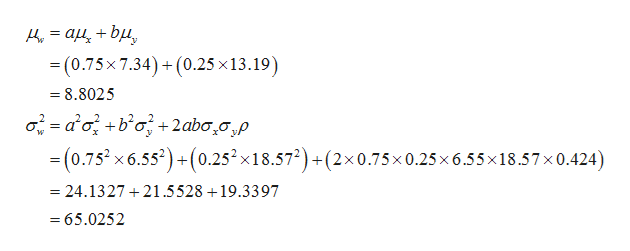Previously, you studied linear combinations of independent random variables. What happens if the variables are not independent? A lot of mathematics can be used to prove the following: Let x and y be random variables with means μx and μy, variances σ2x and σ2y, and population correlation coefficient ρ (the Greek letter rho). Let a and b be any constants and let w = ax + by for the following formula.μw = aμx + bμyσ2w = a2σ2x + b2σ2y + 2abσxσyρIn this formula, r is the population correlation coefficient, theoretically computed using the population of all (x, y) data pairs. The expression σxσyρ is called the covariance of x and y. If x and y are independent, then ρ = 0 and the formula for σ2w reduces to the appropriate formula for independent variables. In most real-world applications the population parameters are not known, so we use sample estimates with the understanding that our conclusions are also estimates.Do you have to be rich to invest in bonds and real estate? No, mutual fund shares are available to you even if you aren't rich. Let x represent annual percentage return (after expenses) on the Vanguard Total Bond Index Fund, and let y represent annual percentage return on the Fidelity Real Estate Investment Fund. Over a long period of time, we have the following population estimates.μx ≈ 7.34,      σx ≈ 6.55,      μy ≈ 13.19,      σy ≈ 18.57,      ρ ≈ 0.424(a) Do you think the variables x and y are independent? Explain your answer.Yes. Interest rates probably has no effect on the investment returns.No. Interest rates probably has no effect on the investment returns.No. Interest rate probably affects both investment returns.Yes. Interest rate probably affects both investment returns.(b) Suppose you decide to put 75% of your investment in bonds and 25% in real estate. This means you will use a weighted average w = 0.75x + 0.25y. Estimate your expected percentage return μw and risk σw.μw =  σw =  (c) Repeat part (b) if w = 0.25x + 0.75y.μw =  σw =  (d) Compare your results in parts (b) and (c). Which investment has the higher expected return? Which has the greater risk as measured by σw?w = 0.75x + 0.25y produces higher return with lower risk as measured by σw.w = 0.25x + 0.75y produces higher return with lower risk as measured by σw.w = 0.25x + 0.75y produces higher return with greater risk as measured by σw.Both investments produce the same return with the same risk as measured by σw.w = 0.75x + 0.25y produces higher return with greater risk as measured by σw.

Question

Previously, you studied linear combinations of independent random variables. What happens if the variables are not independent? A lot of mathematics can be used to prove the following: Let x and y be random variables with means μx and μy, variances σ2x and σ2y, and population correlation coefficient ρ (the Greek letter rho). Let a and b be any constants and let w = ax + by for the following formula.

μw = aμx + bμy
σ2w = a2σ2x + b2σ2y + 2abσxσyρ

In this formula, r is the population correlation coefficient, theoretically computed using the population of all (xy) data pairs. The expression σxσyρ is called the covariance of x and y. If x and y are independent, then ρ = 0 and the formula for σ2w reduces to the appropriate formula for independent variables. In most real-world applications the population parameters are not known, so we use sample estimates with the understanding that our conclusions are also estimates.

Do you have to be rich to invest in bonds and real estate? No, mutual fund shares are available to you even if you aren't rich. Let x represent annual percentage return (after expenses) on the Vanguard Total Bond Index Fund, and let y represent annual percentage return on the Fidelity Real Estate Investment Fund. Over a long period of time, we have the following population estimates.

μx ≈ 7.34,      σx ≈ 6.55,      μy ≈ 13.19,      σy ≈ 18.57,      ρ ≈ 0.424

(a) Do you think the variables x and y are independent? Explain your answer.
Yes. Interest rates probably has no effect on the investment returns.
No. Interest rates probably has no effect on the investment returns.
No. Interest rate probably affects both investment returns.
Yes. Interest rate probably affects both investment returns.

(b) Suppose you decide to put 75% of your investment in bonds and 25% in real estate. This means you will use a weighted average w = 0.75x + 0.25y. Estimate your expected percentage return μw and risk σw.
μw =
σw =

(c) Repeat part (b) if w = 0.25x + 0.75y.
μw =
σw =
(d) Compare your results in parts (b) and (c). Which investment has the higher expected return? Which has the greater risk as measured by σw?
w = 0.75x + 0.25y produces higher return with lower risk as measured by σw.
w = 0.25x + 0.75y produces higher return with lower risk as measured by σw.
w = 0.25x + 0.75y produces higher return with greater risk as measured by σw.
Both investments produce the same return with the same risk as measured by σw.
w = 0.75x + 0.25y produces higher return with greater risk as measured by σw.
Step 1

Hey, since there are multiple subpart questions posted, we will answer first three subparts. If you want any specific subpart to be answered, then please submit that subpart only or specify the subpart number in your message

Step 2

(a) Independence of two variables:

Here, x represent annual percentage return on the Vanguard Total Bond Index Fund and y represent annual percentage return on the Fidelity Real Estate Investment Fund.

If x and y are independent, ρ = 0 and σ2w reduces to the appropriate formula.

The variables x and y are not independent here. Because, the interest rate probably affects both investment returns. The weight of w = ax + by is different from w = bx + ay.

Therefore, the correct answer is No. Interest rate probably affects both investment returns.

Step 3

(b) Estimation of expected percentage return µw and risk σw if w = 0.75x + 0.25y:

It is given that the weighted average w = 0.75x + 0.25y. The estimation of expected percentage return µw is 8....help_outlineImage Transcriptionclose_ (0.75x 7.34)+(0.25 x13.19) 8.8025 02aobo 2aba20,p =(0.752x6.552) +(0.252x18.572) +(2x0.75x0.25x 6.55x18.57x 0.424) + w 24.1327 21.5528 19.3397 65.0252 fullscreen

Want to see the full answer?

See Solution

Want to see this answer and more?

Our solutions are written by experts, many with advanced degrees, and available 24/7

See Solution
Tagged in

Other